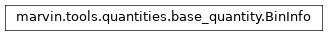# Spaxel¶

## Getting and working with spaxels¶

The basics of spaxels has already been covered in various sections of the Cube or Maps <marvin-maps tools section. We’ll go into a bit more detail here.

### Accessing a Spaxel¶

You can access a spaxel by slicing any `Cube`, `Maps`, or `ModelCube` object. When you slice a tool using array syntax, the spaxel coordinates are numpy array indices, with the `xyorig='lower'`, indicating (0,0) as the lower left.

```>>> from marvin.tools import Cube
>>> cube = Cube('8485-1901')
>>> spaxel = cube[17,17]
>>> spaxel
<Marvin Spaxel (plateifu=8485-1901, x=17, y=17; x_cen=0, y_cen=0, loaded=cube)>
```

Each tool also provides a `getSpaxel` method, which allows access to a `Spaxel` with more fine-grained control. With `getSpaxel`, the x, y spaxel coordinates are by default set to a central origin, with `xyorig='center'`, indicating (0,0) as the spaxel at the center of the IFU. With `xyorig='center'`, positive x is to the right, and positive y is up.

```>>> # access the central spaxel
>>> cube.getSpaxel(x=0, y=0)
<Marvin Spaxel (plateifu=8485-1901, x=17, y=17; x_cen=0, y_cen=0, loaded=cube)>

>>> # access a spaxel 5 pixels north-east from the center
>>> cube.getSpaxel(x=-5,y=5)
<Marvin Spaxel (plateifu=8485-1901, x=12, y=21; x_cen=-5, y_cen=4, loaded=cube)>
```

You can change the `xyorig` as well.

```>>> # access the central spaxel at 0,0
>>> cube.getSpaxel(x=0, y=0)
<Marvin Spaxel (plateifu=8485-1901, x=17, y=17; x_cen=0, y_cen=0, loaded=cube)>
```
```>>> # access the lower left spaxel using array index 0,0
>>> cube.getSpaxel(x=0, y=0, xyorig='lower')
<Marvin Spaxel (plateifu=8485-1901, x=0, y=0; x_cen=-17, y_cen=-17, loaded=cube)>
```

You can also access a spaxel by RA, Dec.

```>>> # access a spaxel by coordinate RA, Dec
>>> cube.getSpaxel(ra=232.543, dec=48.691)
<Marvin Spaxel (plateifu=8485-1901, x=25, y=23; x_cen=8, y_cen=6, loaded=cube)>
```

By default, spaxels automatically load the attributes of the tool from which it is extracted. This is indicated with the loaded keyword in the repr. Spaxels can load `cube`, `maps`, or `modelcube` attributes. A loaded spaxel populates a `{tool}_quantities` attribute containing all the properties relevant for that tool. All attributes in the `{tool}_quantities` are also populated in the objects `__dir__` and available with iPython tab completion feature.

Accessing a spaxel via a `Cube` object only loads `Cube` attributes.

```>>> # accessing via a Cube indicates loaded=cube
>>> from marvin.tools import Cube
>>> cube = Cube('8485-1901')
>>> spaxel = cube[17,17]
<Marvin Spaxel (plateifu=8485-1901, x=17, y=17; x_cen=0, y_cen=0, loaded=cube)>

>>> # access the loaded cube properties
>>> spaxel.cube_quantities
FuzzyDict([('flux',
<Spectrum [0.419787, 0.47158 , 0.430912, ..., 0.      , 0.      , 0.      ] 1e-17 erg / (Angstrom cm2 s spaxel)>),
('dispersion',
<Spectrum [1.09617, 1.09661, 1.09706, ..., 0.     , 0.     , 0.     ] Angstrom>),
('dispersion_prepixel',
<Spectrum [1.05837, 1.05882, 1.05926, ..., 0.     , 0.     , 0.     ] Angstrom>),
('spectral_resolution',
<Spectrum [1414.47, 1414.23, 1413.98, ..., 2013.13, 2013.59, 2014.05] Angstrom>),
('spectral_resolution_prepixel',
<Spectrum [1465.22, 1464.91, 1464.61, ..., 2048.16, 2048.63, 2049.1 ] Angstrom>)])

>>> # try tab completing to access dispersion
>>> spaxel.dispersion
<Spectrum [1.09617, 1.09661, 1.09706, ..., 0.     , 0.     , 0.     ] Angstrom>
```

Accessing from a `Maps` only loads the `Maps` attributes.

```>>> # accessing via a Maps indicates loaded=maps
>>> maps = cube.getMaps()
>>> spaxel = maps[17,17]
<Marvin Spaxel (plateifu=8485-1901, x=17, y=17; x_cen=0, y_cen=0, loaded=maps)>

>>> # access the loaded maps properties
>>> spaxel.maps_quantities
FuzzyDict([('spx_skycoo_on_sky_x', <AnalysisProperty -0.00925397 arcsec>),
('spx_skycoo_on_sky_y', <AnalysisProperty 0.00023976 arcsec>),
<AnalysisProperty 0.0103323 arcsec>),
....])

>>> # notice the empty cube_quantites
>>> spaxel.cube_quantities
FuzzyDict()
```

You can load additional attributes using the `load` method on a spaxel. `load` takes either cube, maps, or modelcube as input. Let’s load the `cube_quantites` from the spaxel in the previous example.

```>>> # load the cube quantities from the maps spaxel
>>> spaxel
<Marvin Spaxel (plateifu=8485-1901, x=17, y=17; x_cen=0, y_cen=0, loaded=cube/maps)>
```

Now loaded is set to `cube/maps` indicating that both quantities are loaded and available.

```>>> spaxel.cube_quantities
FuzzyDict([('flux',
<Spectrum [0.547274, 0.466324, 0.463318, ..., 0.      , 0.      , 0.      ] 1e-17 erg / (Angstrom cm2 s spaxel)>),
('dispersion',
<Spectrum [1.09548, 1.09593, 1.09637, ..., 0.     , 0.     , 0.     ] Angstrom>),
('dispersion_prepixel',
<Spectrum [1.05769, 1.05813, 1.05858, ..., 0.     , 0.     , 0.     ] Angstrom>),
('spectral_resolution',
<Spectrum [1414.47, 1414.23, 1413.98, ..., 2013.13, 2013.59, 2014.05] Angstrom>),
('spectral_resolution_prepixel',
<Spectrum [1465.22, 1464.91, 1464.61, ..., 2048.16, 2048.63, 2049.1 ] Angstrom>)])
```

You can also load multiple attributes when accessing a spaxel with the `getSpaxel` method on tools, by setting either the `cube`, `maps`, or `modelcube` keyword to `True`.

```# load a spaxel from a cube also loading the maps quantities
>>> spaxel = cube.getSpaxel(x=0,y=0, maps=True)
>>> spaxel
<Marvin Spaxel (plateifu=8485-1901, x=17, y=17; x_cen=0, y_cen=0, loaded=cube/maps)>
```

## DataModels¶

Spaxels have both the DRP and DAP datamodels attached, in the `datamodels.drp` and `datamodels.dap` attributes, respectively.

```>>> # access the drp datamodel
>>> spaxel.datamodel.drp
<DRPCubeDataModel release='MPL-7', n_datacubes=3, n_spectra=2>
```

## Working with Bins¶

All `maps` and `modelcube` properties contain a `bin` attribute, providing relevant information about the bin the spaxel belongs to. See the Binning section for more information. Let’s look at the bin info for the central spaxel from the previous example.

```>>> # access the bin info for stellar_velocity
>>> stvel = spaxel.stellar_vel
>>> stvel.bin
<BinInfo (binid=0, n_spaxels=1)>
```

The central spaxel has a binid of 0, with this spaxel the only one belonging in that bin. Let’s look at the bin information for H-alpha flux. This bin also only has one spaxel in it.

```>>> spaxel.emline_gflux_ha_6564.bin
<BinInfo (binid=199, n_spaxels=1)>
```

The `BinInfo` also provides a convenience method, `get_bin_spaxels`, for getting all spaxels belonging to that bin. These spaxels are unloaded by default.

```>>> stvel.bin.get_bin_spaxels()
[<Marvin Spaxel (x=17, y=17, loaded=False)]
```

## Reference/API¶

Class Inheritance DiagramClass

 `marvin.tools.spaxel.Spaxel`(x, y[, cube, …]) A base class that contains information about a spaxel.

Methods

 Returns the associated `Cube` Returns the associated `Maps` Returns the associated `ModelCube` `marvin.tools.spaxel.Spaxel.save`(path[, …]) Pickles the spaxel to a file. `marvin.tools.spaxel.Spaxel.restore`(path[, …]) Restores a Spaxel object from a pickled file.